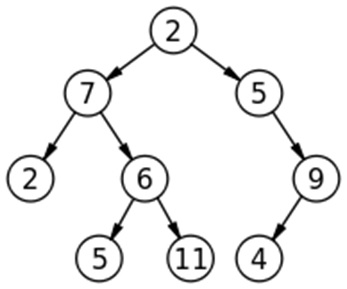# C program to convert a Binary Tree into a Singly Linked List by Traversing Level by Level

In this article, we are going to see how to convert a binary tree into a single link list by traversing level-wise?
Submitted by Radib Kar, on January 07, 2019

Problem statement: Write a C program to convert a binary tree into a single linked list by traversing level-wise.

Example:The above binary tree is converted to 2 → 7 → 5 → 2 → 6 → 9 → 5 → 11 → 4 → NULL

Solution

1. Building a linked list - Setting the first node as Head and then appending other nodes.
2. Traversing & displaying a single link list.
3. Building a tree & level order traversal of a tree.

Algorithm:

1. Assign the root value as head node value in linked list.
2. Do level-order traversal
For each tree node create a single linked list node and append it to the linked list.
3. Display the single linked list.

How the tree is converted to the single list...

Let’s do solve the above example.

```Root=2;
Queue status: 2
----------------------------------------------------

1st iteration
Queue not empty
Queue front is 2
Pop 2
Push: 2->left(7) & 2->right(5)
Queue status: 7, 5
----------------------------------------------------

2nd iteration
Queue not empty
Queue front is 7
Head is not null, thus append 7
Pop 7
Push: 7->left(2)& 7->right(6)
Queue status: 5, 2, 6
----------------------------------------------------

3rd iteration
Queue not empty
Queue front is 5
Head is not null, thus append 5
Pop 5
Push: 5->right (9) only (5->left is NULL)
Queue status: 2, 6, 9
----------------------------------------------------

4th iteration
Queue not empty
Queue front is 2
Head is not null, thus append 2
Pop 2
Push: Nothing ( both child are NULL)
Queue status: 6, 9
----------------------------------------------------

5th iteration
Queue not empty
Queue front is 6
Head is not null, thus append 6
Pop 6
Push: 6->left(5) and 6->right(11)
Queue status: 9, 5, 11
----------------------------------------------------

6th iteration
Queue not empty
Queue front is 9
Head is not null, thus append 9
Pop 9
Push: 9->left(4) only (right child NULL)
Queue status: 5, 11, 4
----------------------------------------------------

7th iteration
Queue not empty
Queue front is 5
Head is not null, thus append 5
Pop 5
Push: Nothing (both child NULL)
Queue status: 11, 4
----------------------------------------------------

8th iteration
Queue not empty
Queue front is 11
Head is not null, thus append 11
Pop 11
Push: Nothing (both child NULL)
Queue status: 4
----------------------------------------------------

8th iteration
Queue not empty
Queue front is 4
Head is not null, thus append 4
Linked list up to now: 2->7->5->2->6->9->5->11->4->NULL
Pop 4
Push: Nothing (both child NULL)
Queue status: empty queue
----------------------------------------------------

Iteration stops
So final link list is: 2->7->5->2->6->9->5->11->4->NULL
```

## C implementation to to convert a Binary Tree into a Singly Linked List by Traversing Level by Level

```#include <bits/stdc++.h>
using namespace std;

// tree node is defined
class tree{
public:
int data;
tree *left;
tree *right;
};

class sll{
public:
int data;
sll* next;
};

sll* creatnode(int d){ //create node for single linked list
sll* temp=(sll*)malloc(sizeof(sll));
temp->data=d;
temp->next=NULL;
return temp;
}

printf("displayig the converted list...\n");
while(current!=NULL){ //traverse until current node isn't NULL
if(current->next)
printf("%d->",current->data);
else
printf("%d->NULL\n",current->data);
current=current->next; // go to next node
}
}

sll* flatten(tree* root)
{
//Declare queue using STL
queue<tree*> q;
//enqueue the root
q.push(root);
vector<int> store;

tree* temp;
//do the level order traversal & build single linked list
while(!q.empty()){
//dequeue
temp=q.front();
q.pop();
}
else{//for inserting rest of the nodes
tempL->next=creatnode(temp->data);
tempL=tempL->next;
}

// do level order traversing
if(temp->left)//for left child
q.push(temp->left);
if(temp->right)//for right child
q.push(temp->right);

}

}

tree* newnode(int data)  // creating new node for tree
{
tree* node = (tree*)malloc(sizeof(tree));
node->data = data;
node->left = NULL;
node->right = NULL;

return(node);
}

int main()
{
//**same tree is builted as shown in example**
cout<<"same tree is built as shown in example\n";
tree *root=newnode(2);
root->left= newnode(7);
root->right= newnode(5);
root->right->right=newnode(9);
root->right->right->left=newnode(4);
root->left->left=newnode(2);
root->left->right=newnode(6);
root->left->right->left=newnode(5);
root->left->right->right=newnode(11);

cout<<"converting the tree into a single link list...\n";
cout<<"by traversing the tree level-wise\n";
//displaying the list built from the tree

return 0;
}
```

Output

```same tree is built as shown in example
converting the tree into a single link list...
by traversing the tree level-wise
displayig the converted list...
2->7->5->2->6->9->5->11->4->NULL
```